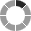Eonza automation software - free, open source cross-platform program for easy creation and management of scripts.

# Calculated fields and formula expression rules

Let’s first deal with what are calculated fields and what purpose they serve. As seen from the name, this field type isn’t stored in the table but instead is calculated dynamically whenever a query fetches data from the database. The value is calculated for every record using the formula specified in the description. Let’s say you have a table with three columns each storing the number of kilocalories eaten on breakfast, dinner and supper respectively. To calculate overall kilocalories per day you simply add a calculated field with the following formula [kcal1] + [kcal2] + [kcal3],where each alias specifies the corresponding source field.

You can add multiple calculated fields to your table. Pay attention when composing formulas and follow the below rules otherwise you may end up with your table not displayed at all. If you encounter an error, try gradually simplifying the formula until you locate the source of the error.

## Composing formulas

1. You can only specify numeric fields in the formula having either the Number or Decimal number type and enclose these fields in square brackets. You should use the alias of the field as a name here or the id of the column if the alias is missing.

2. Formulas support basic mathematic expressions including addition, subtraction, multiplying, and division. You can use parentheses to set the order of evaluation.

```( [count] +  )/10
[count]*[price]
( [count]* - [val1] )/365```
3. You can use the following functions in formula expressions:

• abs(X) - returns absolute value of the number.
• mod(N,M) - returns the remainder after division N by M.
• floor(X) - rounds the argument down.
• ceiling(X) - rounds the argument up.
• round(X) - rounds the argument to the nearest integer.
• round(X,D) - rounds the argument to the number with D decimal digits. If D is 0, the result is returned without the fractional part.
• truncate(X,D) - returns the number truncated down to D decimal digits.
```floor( [myfield1]/7 )
[par1] + truncate([par2]) - ```
4. Take into account that if you multiply columns, they shouldn’t take negative values. If some column may contain negative values, apply the abs function.

`[myfield1]*abs([myfield2])`
5. To round numbers you can use the Round parameter in the field settings. The parameter specifies the number of decimal digits.